The preferred analysis and graphing solution purpose-built for scientific research. Join the world’s leading scientists and discover how you can use Prism to save time, make more appropriate analysis choices, and elegantly graph and present your scientific research.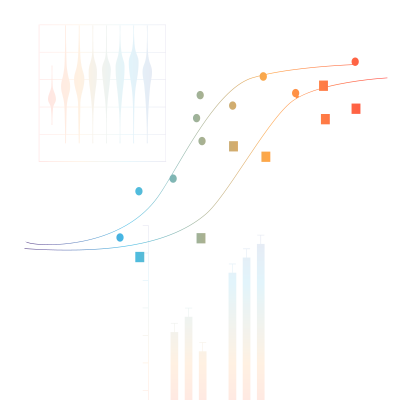## Comprehensive Analysis and Powerful Statistics, Simplified

Prism is specifically formatted for the analyses you want to run, including analysis of quantitative and categorical data. This makes it easier to enter data correctly, choose suitable analyses, and create stunning graphs.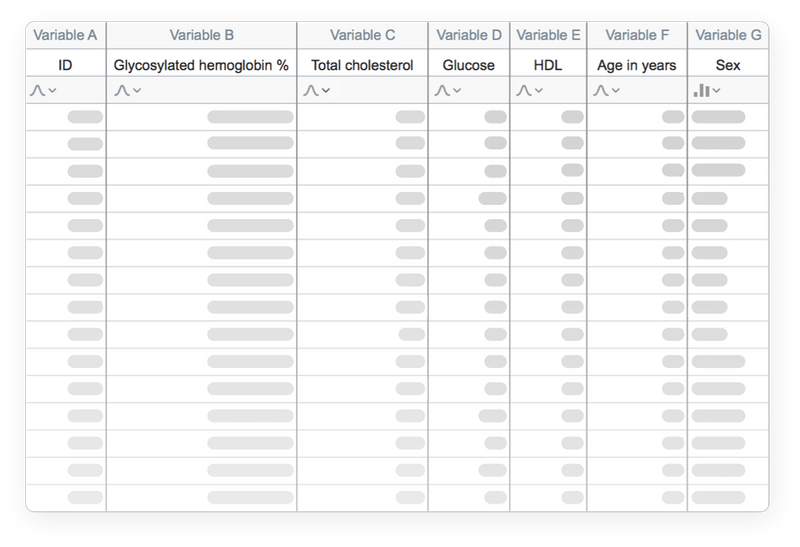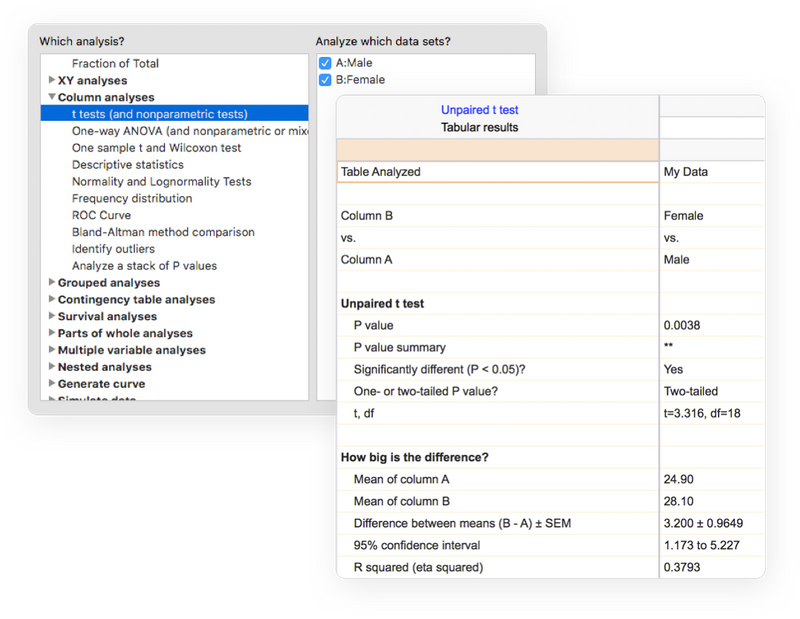### Perform The Right Analysis

Avoid statistical jargon. In clear language, Prism presents an extensive library of analyses from common to highly specific— t tests, one-, two- and three-way ANOVA, linear and nonlinear regression, dose-response curves, binary logistic regression, survival analysis, principal component analysis, and much more. Each analysis has a checklist to help you understand the required statistical assumptions and confirm you have selected an appropriate test.

### Get Actionable Help As You Go

Reduce the complexity of statistics. Prism’s online help goes beyond your expectations. At almost every step, access thousands of pages from the Prism User Guides. Learn from video lessons, guides and educational materials through Prism Academy. Browse the Graph Portfolio and learn how to make a wide range of graph types. Tutorial data sets also help you understand why you should perform certain analyses and how to interpret your results.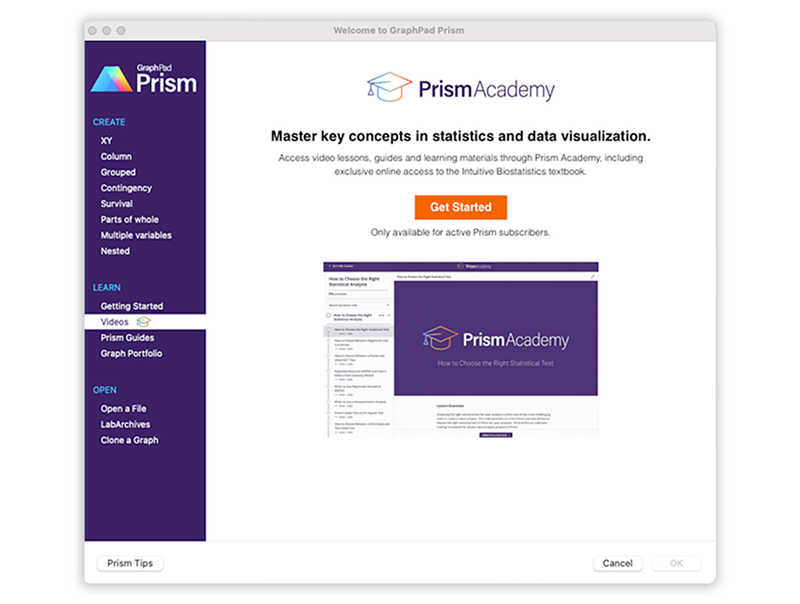## Work Smarter, Not Harder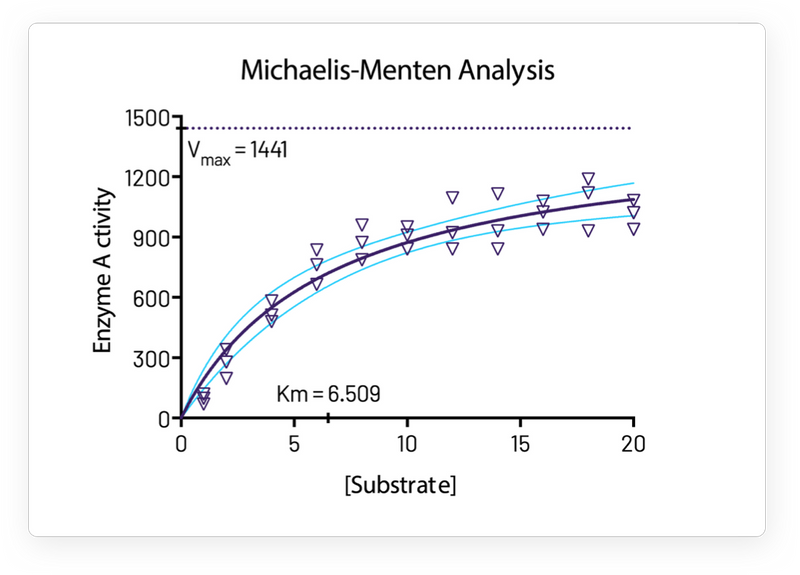### One-Click Regression Analysis

No other program simplifies curve fitting like Prism. Select an equation and Prism does the rest—fits the curve, displays a table of results and function parameters, draws the curve on the graph, and interpolates unknown values.

Prism handles the coding for you. Graphs and results are automatically updated in real time. Any changes to the data and analyses— direct entry of data, omitting erroneous data, correcting typos, or changing analysis choices—are reflected in results, graphs, and layouts instantaneously.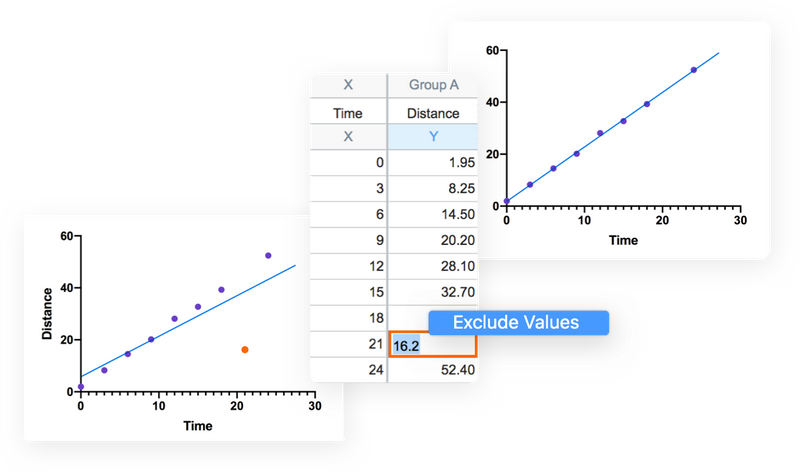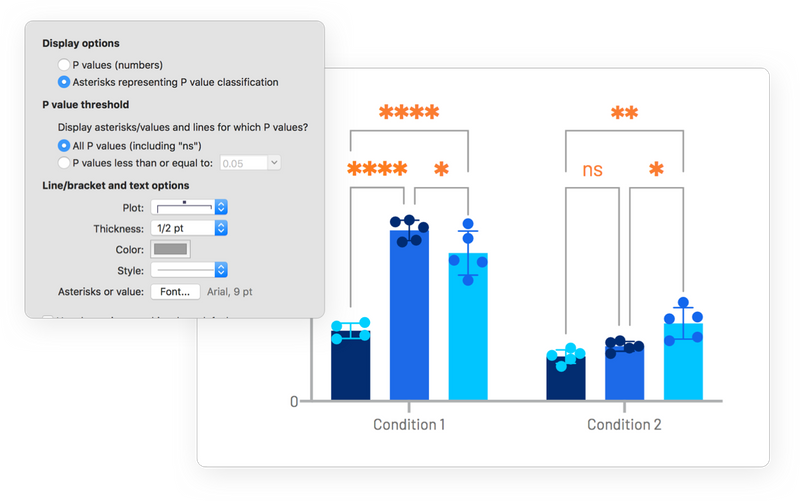### Automate Your Work Without Programming

Automatically add multiple pairwise comparisons to your analysis with a single click. For customization options of these lines and asterisks, simply click the toolbar button again. Make adjustments to the data or the analysis, and the results displayed on the graph will update automatically.

## The Fastest Way to Elegantly Graph and Share Your Work

### Countless Ways to Customize Your Graphs

Explore countless ways to present a single data set. Select the visualization style that most clearly tells the story contained within your data. Simply choose a graph type, then customize it in real-time with changes to how the data are arranged, the style of the data points, labels, fonts, colors, and much more. The customization options are endless.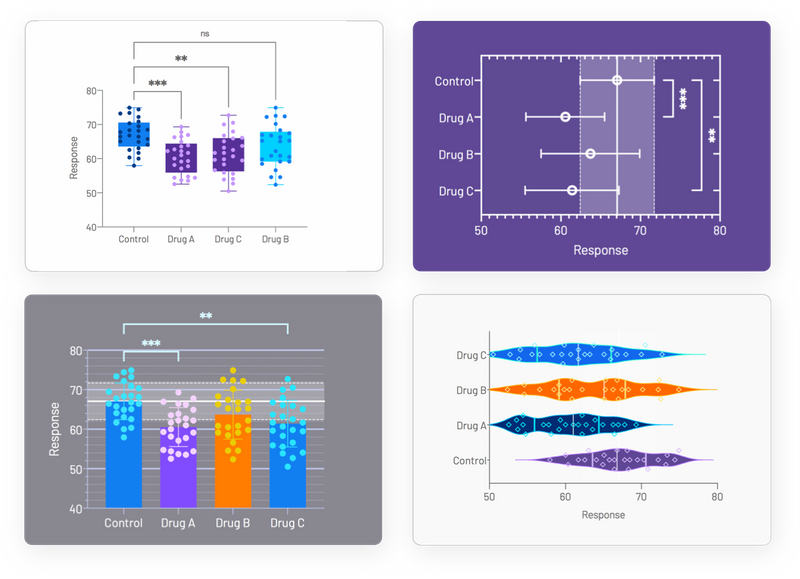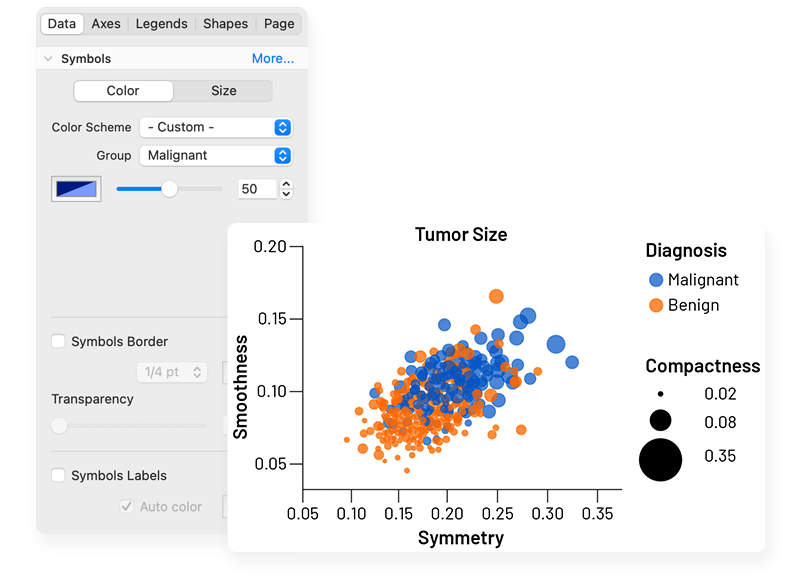Focus your efforts on analyzing the most relevant data. Customize how you present the relationships in the data to effectively explore large data sets. Notice something interesting? Highlight a single data point to explore its corresponding properties. With Prisms' robust data wrangling capabilities, you can both save time and ensure that your analysis is based on clean and well-structured data.

### Export Publication-Quality Graphs With One Click

Reduce time to publish. Prism allows you to customize your exports (file type, resolution, transparency, dimensions, color space RGB/CMYK) to meet the requirements of journals. Set your defaults to save time.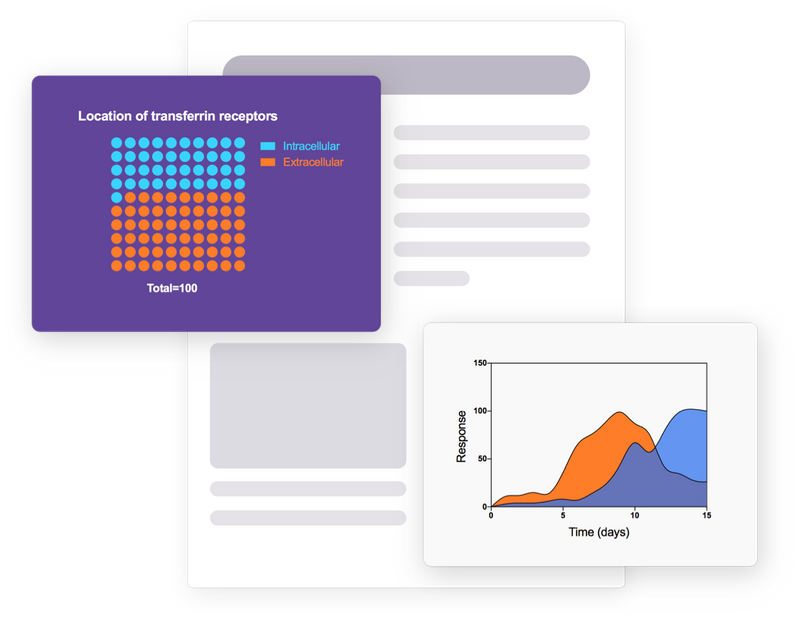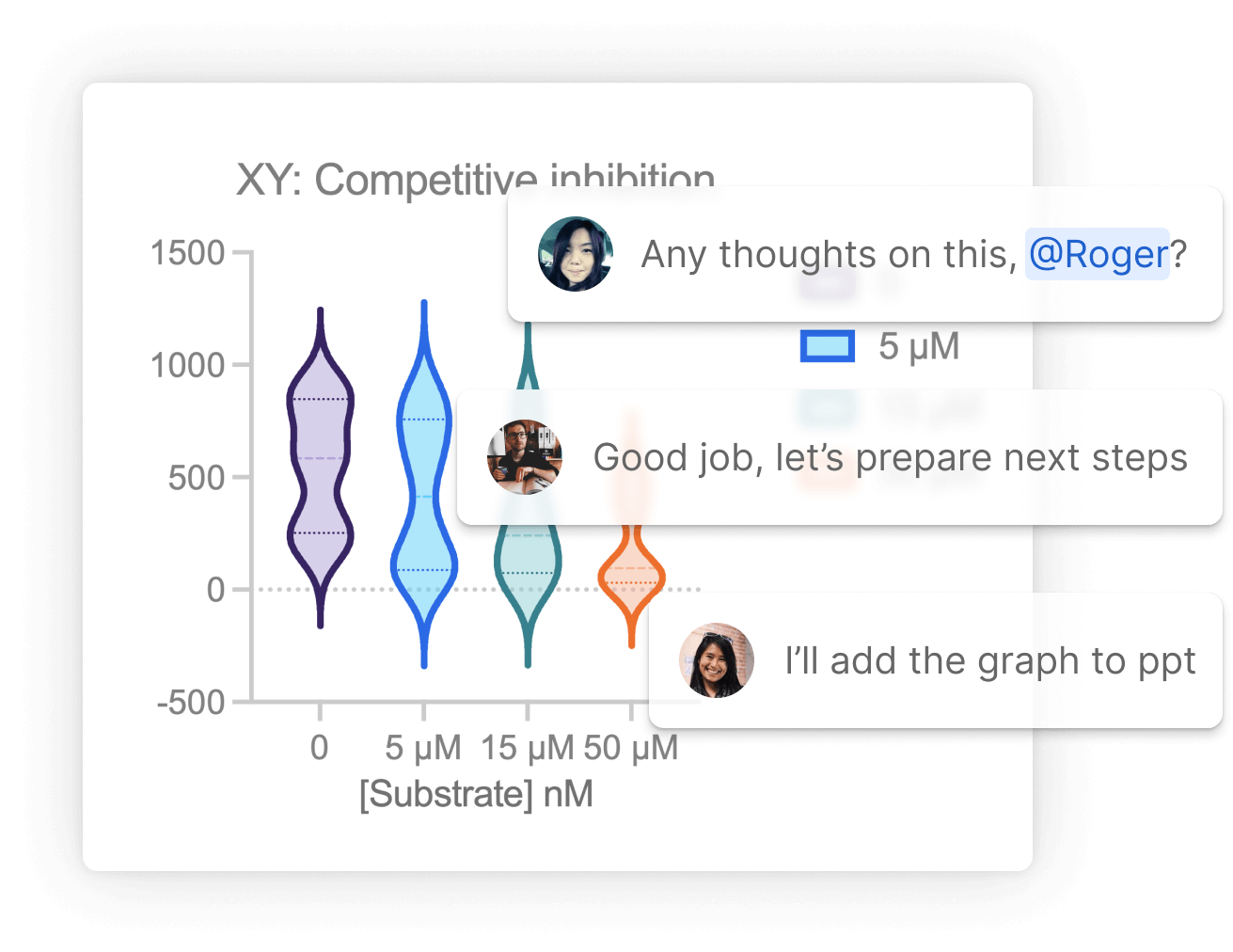### Collaboration. Simplified.

Avoid those messy email threads and use Prism Cloud to share, view and collaborate on your Prism projects. Safeguard the interoperability and reusability of your results with Prism’s open access file format. By using industry-standard formats (CSV, PNG, JSON, etc.), you can ensure that your projects can be utilized outside of Prism to open up new possibilities for your data workflows and integrations.

## Discover What’s New in Prism!

Introducing an even more powerful Prism featuring enhanced data visualization and graph customization, more intuitive navigation, and more sophisticated statistical analyses.

### Enhanced Data Visualization and Graphs Customization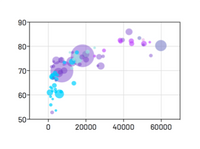#### Bubble Plots

Create Bubble Plots directly from raw data, encoding variables for position (X- and Y-coordinates), color, and size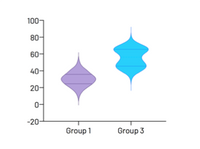#### Violin plots

Visualize distributions of large data sets with either extended or truncated violin plots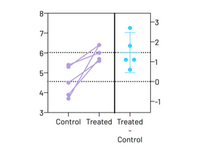#### Estimation Plots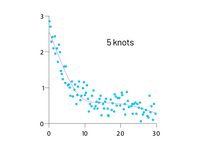#### Smoothing spline

Major improvements in showing general data trends through Akima splines and smoothing splines with improved control over the number of knots, or inflection points

### Improved Graphing and Customization Options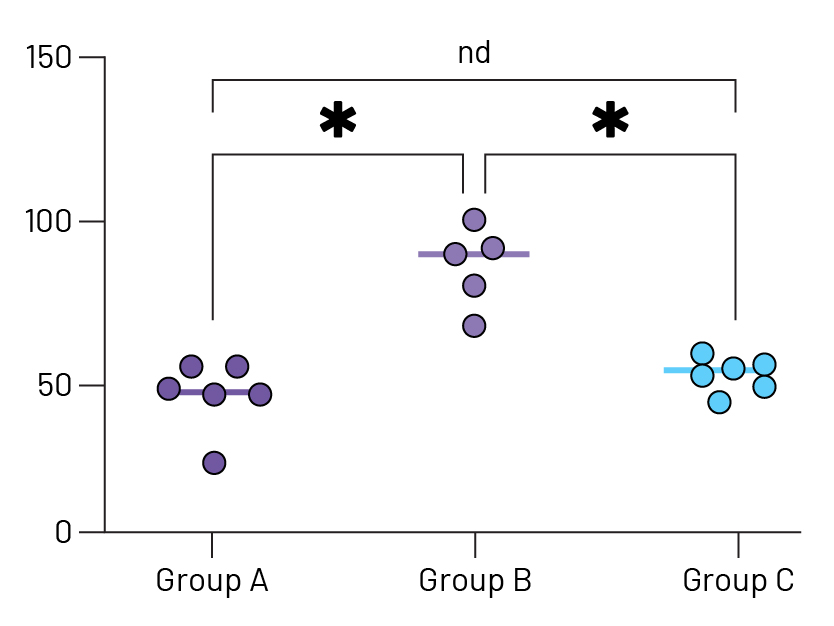#### Stars on Graph

Automatically add multiple comparison results to graphs. Choose from a variety of P value summary styles including a responsive method appropriate for any alpha level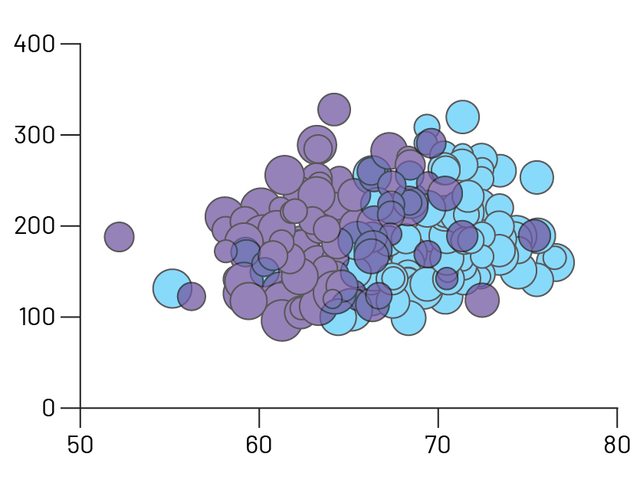#### Improved Graph Customization

Craft stunning Bubble plots faster, easier, and more intuitively than before. Interact and customize graphs from your Multiple Variables data in real-time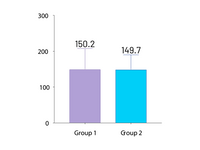#### Automatically label bar graphs

Annotate your bar graphs with values for the means, medians, or sample sizes to emphasize what's important in your work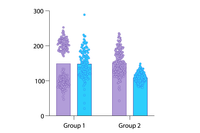#### Improved grouped graphs

Easily create graphs that show both individual points (scatter) along with bars for mean (or median) and error bars

### A More Open Access File Format

By using industry-standard formats (CSV, PNG, JSON, etc.), you can ensure that your projects can be utilized outside of Prism and open up new possibilities for your data workflows and integrations

### Expanded Data Table Capabilities

You can have as many windows open as you need and data in up to 2048 columns, with 512 sub columns in each. Expanded analysis constant dialog allows you to link to more results for all types of analyses

### Intelligent Data Wrangling

A new range of tools to help prepare your data for analysis. Covering multiple variables data tables, select and transform analysis and extract and rearrange functionality

A convenient way to establish connections between different elements in Prism. A new easy-to-navigate tree structure now covers the entire library of Prism analyses

### XY tables

Used when every data point is defined by a single X and Y value. These sorts of data are often fit with linear or nonlinear regression models.

### Column tables

Used for data organized into groups by a single grouping variable (for example Treatment vs. Control or Female vs. Male). Often analyzed using t tests and one-way ANOVA.

### Grouped tables

Used for data organized into groups by two grouping variables (for example Female Control vs. Female Treatment vs. Male Control vs. Male Treatment). Often analyzed using two-way ANOVA.

### Contingency tables

Used for count data organized into groups by two grouping variables (Treatment vs. Control and Positive vs. Negative Outcome). Appropriate for analysis by Chi-square and Fisher's exact test.

### Survival tables

Used to perform Kaplan-Meier survival analysis. Each row represents a subject or individual with elapsed survival time and outcome.

### Parts of whole tables

Used when it makes sense to ask: "What fraction of the total is each value in the table?". Used for calculating fractions and generating pie charts.

### Multiple variables tables

Used when each row of the data is a different observation and each column is a different variable and supports text values. Can be analyzed directly with multiple linear regression, Cox regression, and others or restructured into one of Prism's other table types.

### Nested tables

Used when the data are organized into hierarchical groups. Analyzed using nested t tests or nested one-way ANOVA.

### Perform repeated measures ANOVA – even with missing data

Now Prism will automatically fit a mixed effects model to complete this analysis

### Perform simple and multiple logistic regression

Fit a model to a binary outcome (yes/no, win/lose, pass/fail) based on one predictor variable (simple logistic regression) or many predictor variables (multiple logistic regression)

### Principal Component Analysis (PCA)

Calculate and select the principal components that describe the greatest amount of variance in your data. Choose from selection techniques including Parallel Analysis via Monte Carlo simulation, Eigenvalue threshold, Proportion of Variance threshold, and more

### Multiple t test (and nonparametric) analyses

Perform multiple independent two-sample comparison tests simultaneously. Select from parametric/nonparametric tests, and specify if data are unpaired/paired

### Analyze categorical variables with text in Multiple Linear and Multiple Logistic Regression

No coding required! Prism will automatically encode categorical variables and perform the analysis. Specify a reference and organize all levels of categorical variables in a model for clear, readable results

### Interpolation from multiple linear and multiple logistic regression

Use the specified model to predict values for the dependent variable based on data in the data table or using theoretical values specified in the analysis

No credit card required.

## Discover the Breadth of Statistical Features Available in Prism

#### Statistical Comparisons

• Paired or unpaired t tests. Reports P values and confidence intervals.
• Automatically generate volcano plot (difference vs. P value) from multiple t test analysis.
• Nonparametric Mann-Whitney test, including confidence interval of difference of medians.
• Kolmogorov-Smirnov test to compare two groups.
• Wilcoxon test with confidence interval of median.
• Perform many t tests at once, using False Discovery Rate (or Bonferroni multiple comparisons) to choose which comparisons are discoveries to study further.
• Ordinary or repeated measures ANOVA followed by the Tukey, Newman-Keuls, Dunnett, Bonferroni or Holm-Sidak multiple comparison tests, the post-test for trend, or Fisher’s Least Significant tests.
• One-way ANOVA without assuming populations with equal standard deviations using Brown-Forsythe and Welch ANOVA, followed by appropriate comparisons tests (Games-Howell, Tamhane T2, Dunnett T3)
• Many multiple comparisons test are accompanied by confidence intervals and multiplicity adjusted P values.
• Greenhouse-Geisser correction so repeated measures one-, two-, and three-way ANOVA do not have to assume sphericity. When this is chosen, multiple comparison tests also do not assume sphericity.
• Kruskal-Wallis or Friedman nonparametric one-way ANOVA with Dunn's post test.
• Fisher's exact test or the chi-square test. Calculate the relative risk and odds ratio with confidence intervals.
• Two-way ANOVA, even with missing values with some post tests.
• Two-way ANOVA, with repeated measures in one or both factors. Tukey, Newman-Keuls, Dunnett, Bonferroni, Holm-Sidak, or Fisher’s LSD multiple comparisons testing main and simple effects.
• Three-way ANOVA (limited to two levels in two of the factors, and any number of levels in the third).
• Analysis of repeated measures data (one-, two-, and three-way) using a mixed effects model (similar to repeated measures ANOVA, but capable of handling missing data).
• Comparison of data from nested data tables using nested t test or nested one-way ANOVA (using mixed effects model).

#### Nonlinear Regression

• Fit one of our 105 built-in equations, or enter your own. Now including family of growth equations: exponential growth, exponential plateau, Gompertz, logistic, and beta (growth and then decay).
• Enter differential or implicit equations.
• Enter different equations for different data sets.
• Global nonlinear regression – share parameters between data sets.
• Robust nonlinear regression.
• Automatic outlier identification or elimination.
• Compare models using extra sum-of-squares F test or AICc
• Compare parameters between data sets.
• Apply constraints.
• Differentially weight points by several methods and assess how well your weighting method worked.
• Accept automatic initial estimated values or enter your own.
• Automatically graph curve over specified range of X values.
• Quantify precision of fits with SE or CI of parameters. Confidence intervals can be symmetrical (as is traditional) or asymmetrical (which is more accurate).
• Quantify symmetry of imprecision with Hougaard’s skewness.
• Plot confidence or prediction bands.
• Test normality of residuals.
• Runs or replicates test of adequacy of model.
• Report the covariance matrix or set of dependencies.
• Easily interpolate points from the best fit curve.
• Fit straight lines to two data sets and determine the intersection point and both slopes.

#### Survival Analysis

• Kaplan-Meier survival analysis. Perform nonparametric survival analysis for different groups, and compare the estimated survival curves for each group with the log-rank test (including test for trend).
• Cox proportional hazards regression. Perform semi-parametric survival analysis that allows for the inclusion of additional continuous or categorical predictor variables (covariates). Automatically generate graphs of estimated survival curves for any set of predictor variable values.

#### Principal Component Analysis

• Component selection via Parallel Analysis (Monte Carlo simulation), Kaiser criterion (Eigenvalue threshold), Proportion of Variance threshold, and more.
• Use results in downstream applications like Principal Component Regression.

#### Multiple Variable Graphing

• Specify variables defining axis coordinates, color, and size.
• Create Bubble Plots.

#### Column Statistics

• Calculate descriptive statistics: min, max, quartiles, mean, SD, SEM, CI, CV, skewness, kurtosis.
• Mean or geometric mean with confidence intervals.
• Frequency distributions (bin to histogram), including cumulative histograms
• Normality testing by four methods (new: Anderson-Darling).
• Lognormality test and likelihood of sampling from normal (Gaussian) vs. lognormal distribution
• Create QQ Plot as part of normality testing.
• One sample t test or Wilcoxon test to compare the column mean (or median) with a theoretical value.
• Identify outliers using Grubbs or ROUT method.
• Analyze a stack of P values, using Bonferroni multiple comparisons or the FDR approach to identify "significant" findings or discoveries.

#### Simple Linear Regression and Correlation

• Calculate slope and intercept with confidence intervals.
• Force the regression line through a specified point.
• Fit to replicate Y values or mean Y.
• Test for departure from linearity with a runs test.
• Calculate and graph residuals in four different ways (including QQ plot).
• Compare slopes and intercepts of two or more regression lines.
• Interpolate new points along the standard curve.
• Pearson or Spearman (nonparametric) correlation.

#### Generalized Linear Models (GLMs)

• Generate models relating multiple independent variables to a single dependent variable using the new multiple variables data table.
• Multiple linear regression (when Y is continuous).
• Poisson regression (when Y is counts; 0, 1, 2, …).
• Logistic regression (when Y is binary; yes/no, pass/fail, etc.).

#### Clinical (Diagnostic) Lab Statistics

• Bland-Altman plots.
• Receiver operator characteristic (ROC) curves.
• Deming regression (type ll linear regression).

#### Simulations

• Simulate XY, Column or Contingency tables.
• Repeat analyses of simulated data as a Monte-Carlo analysis.
• Plot functions from equations you select or enter and parameter values you choose.

#### Other Calculations

• Area under the curve, with confidence interval.
• Transform data.
• Normalize.
• Identify outliers.
• Normality tests.
• Transpose tables.
• Subtract baseline (and combine columns).
• Compute each value as a fraction of its row, column or grand total.

Prism is available on Mac and Windows.